# AKT-09/HW3

(diff) ← Older revision | Latest revision (diff) | Newer revision → (diff)
Problem 1 With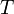$T$,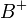$B^+$ and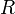$R$ as below, write$B^+$ as a composition of$T$ and two$R$'s, using he basic TG operations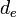$d_e$,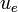$u_e$, and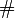$\#$.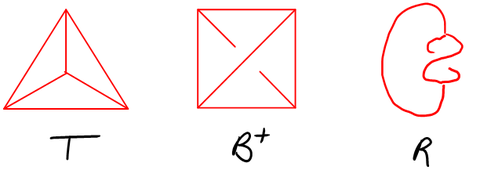Problem 2 Show that the "topological boundary" operator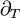$\partial_T$ and the "crossing change" operator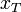$x_T$ of the class of November 5 are compositions of the basic TG operations$d_e$,$u_e$, and$\#$ (you are also allowed to use "nullary" operations, otherwise known as "constants").
Problem 3 Write the third Reidemeister move R3 as a relation on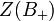$Z(B_+)$.